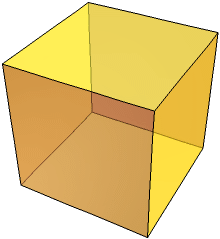Search IntMath
Close

450+ Math Lessons written by Math Professors and Teachers

5 Million+ Students Helped Each Year

1200+ Articles Written by Math Educators and Enthusiasts

Simplifying and Teaching Math for Over 23 Years

# Understanding the Difference between Cube and Cuboid in Geometry## What is Geometry?

Geometry is a branch of mathematics that deals with shapes, sizes, and relative position of figures and with properties of space. Geometry focuses on objects such as points, lines, planes, and shapes.

## What are 3D Shapes?

3D shapes, also known as three-dimensional shapes, are shapes that have three dimensions � length, width, and height. The most common 3D shapes are cubes and cuboids, but there are also many other 3D shapes such as cylinders, cones, pyramids, and spheres.

## What is a Cube?

A cube is a three-dimensional shape with six equal faces and all angles equal to 90 degrees. It is one of the five Platonic solids and is also known as a regular hexahedron. A cube has 12 edges, 8 vertices, and 6 faces, and all of its sides are the same length. The volume of a cube is calculated by cubing the length of one of its sides.

## What is a Cuboid?

A cuboid is a three-dimensional shape with six faces and all angles equal to 90 degrees. It is also known as a rectangular prism. A cuboid has 12 edges, 8 vertices, and 6 faces, but the length of its sides can be different. The volume of a cuboid is calculated by multiplying the length, width, and height.

## How Do You Tell the Difference Between a Cube and a Cuboid?

The main difference between a cube and a cuboid is that a cube has all its sides the same length, while a cuboid has sides of different lengths. Furthermore, the volume of a cube is calculated by cubing the length of one of its sides, while the volume of a cuboid is calculated by multiplying the length, width, and height.

## Practice Problems

1. What is the volume of a cube with sides of length 5 cm?
Answer: 125 cm3 (53 = 125 cm3)

2. What is the volume of a cuboid with length 10 cm, width 5 cm, and height 3 cm?
Answer: 150 cm3 (10 x 5 x 3 = 150 cm3)

3. What are the three dimensions of a cube?

4. What is the difference between a cube and a cuboid?
Answer: The main difference between a cube and a cuboid is that a cube has all its sides the same length, while a cuboid has sides of different lengths.

5. How is the volume of a cube calculated?
Answer: The volume of a cube is calculated by cubing the length of one of its sides.

6. How is the volume of a cuboid calculated?
Answer: The volume of a cuboid is calculated by multiplying the length, width, and height.

## Summary

In this article, we explored the difference between cube and cuboid in geometry. We discussed what geometry is and what 3D shapes are. We then discussed what a cube and a cuboid are, and how to tell the difference between them. We also looked at how the volume of each shape is calculated and provided some practice problems with answers. Understanding the difference between cube and cuboid is important for anyone studying geometry.

## FAQ

### What is the difference between a cube and a cuboid?

The main difference between a cube and a cuboid is that a cube has six equal sides and all the angles are 90 degrees, whereas a cuboid has six sides that are not all equal and the angles are not all 90 degrees.

### How many faces does a cube have?

A cube has six faces.